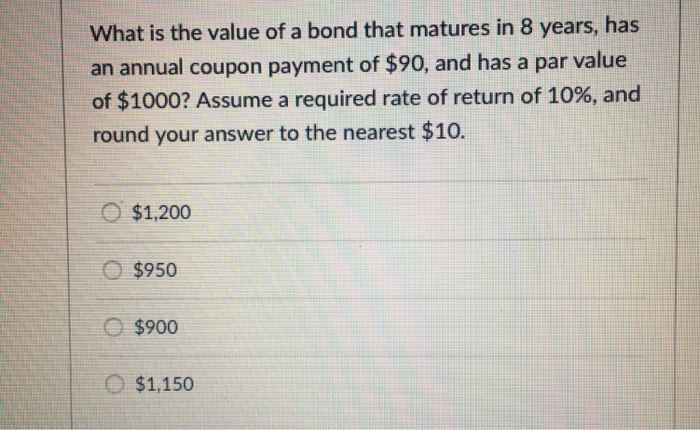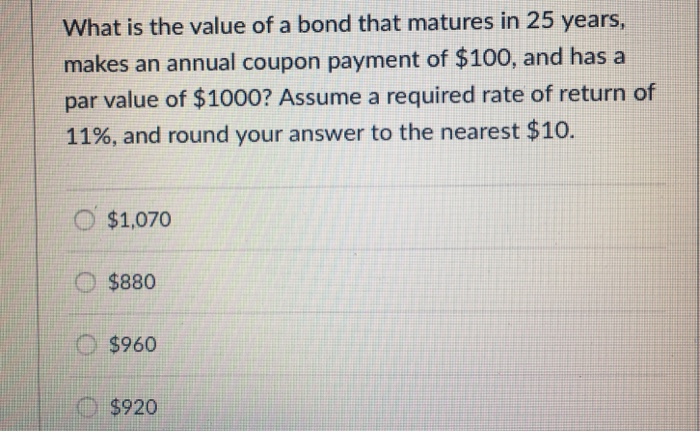Question

What is the value of a bond that matures in 8 years, has an annual coupon...What is the value of a bond that matures in 8 years, has an annual coupon payment of \$90, and has a par value of \$1000? Assume a required rate of return of 10%, and round your answer to the nearest \$10. O \$1,200 \$950 O \$900 O \$1,150

Value of bond=Annual coupon*Present value of annuity factor(10%,8)+\$1000*Present value of discounting factor(10%,8)

=\$90*5.334926198+\$1000*0.46650738

=\$950(Approx).

NOTE:

1.Present value of annuity=Annuity[1-(1+interest rate)^-time period]/rate

=\$90[1-(1.1)^-8]/0.1

=\$90*5.334926198

2.Present value of discounting factor=\$1000/1.1^8

=\$1000*0.46650738

Earn Coins

Coins can be redeemed for fabulous gifts.

Similar Homework Help Questions
• What is the value of a bond that matures in 25 years, makes an annual coupon...What is the value of a bond that matures in 25 years, makes an annual coupon payment of \$100, and has a par value of \$1000? Assume a required rate of return of 11%, and round your answer to the nearest \$10. O \$1,070 \$880 O \$960 \$920

• 1. a corperate bond matures in 3 years. the bond has an 8% semiannual coupon and...

1. a corperate bond matures in 3 years. the bond has an 8% semiannual coupon and the par value is 1000. the bond is callable in 2 years at a call price of \$1050. the price of the bond today is \$1075. what is the bonds yield to call? 2. midea cooperation bonds mature in 3 years and have a yield to maturity of 8.5%. the par value is 1000. the bond has a 10% coupon rate and pay interest...

• A coupon bond that matures in 3 years paying an annual coupon of 8% (in semi-annual...

A coupon bond that matures in 3 years paying an annual coupon of 8% (in semi-annual installments) with a face value of \$1000 has an annual yield-to-maturity of 6%. The bond made its most recent interest payment yesterday, and so has 6 interest payments remaining. What is the price of the bond? Round the price to two decimal places (i.e. 123.45).

• You own a bond that has a 6% annual coupon rate and matures 5 years from...You own a bond that has a 6% annual coupon rate and matures 5 years from now. You purchased this 10-year bond at par value when it was originally issued. Which one of the following statements applies to this bond if the relevant market interest rate is now 5.8% (yield to maturity)? You purchase a bond with a coupon rate of 6.25% and a par value of \$1,000. There are 53 days to the next semiannual coupon payment date and...

• A bond that matures in 17 years has a ​\$1000 par value. The annual coupon interest...

A bond that matures in 17 years has a ​\$1000 par value. The annual coupon interest rate is 15 percent and the​ market's required yield to maturity on a​ comparable-risk bond is 14 percent. What would be the value of this bond if it paid interest​ annually? What would be the value of this bond if it paid interest​ semiannually? Question 7-3

• IBM issues an 11% annual coupon rate bond that matures in 16 years. The face value...

IBM issues an 11% annual coupon rate bond that matures in 16 years. The face value is \$1000. The required rate of return on bonds of similar risk and maturity is 9%. 1. Is this IBM bond selling at a premium, at par or at a discount and explain why? 2. What is the price of this bond and show how? 3. Coupon payments are made monthly. What is the price of THIS bond and show how you got your...

• A bond that matures in 13 years has a ​\$1,000 par value. The annual coupon interest...

A bond that matures in 13 years has a ​\$1,000 par value. The annual coupon interest rate is 8 percent and the​ market's required yield to maturity on a​ comparable-risk bond is 16 percent. What would be the value of this bond if it paid interest​ annually? What would be the value of this bond if it paid interest​ semiannually?

• ​(Bond valuation) A bond that matures in 19 years has a ​\$1,000 par value. The annual...

​(Bond valuation) A bond that matures in 19 years has a ​\$1,000 par value. The annual coupon interest rate is 14 percent and the​ market's required yield to maturity on a​ comparable-risk bond is 13 percent. What would be the value of this bond if it paid interest​ annually? What would be the value of this bond if it paid interest​ semiannually? a.  The value of this bond if it paid interest annually would be ​\$ nothing. ​(Round to the...

• A bond that matures in 12 years has a \$1,000 par value. The annual coupon interest rate is 11 percent and the​ market's...

A bond that matures in 12 years has a \$1,000 par value. The annual coupon interest rate is 11 percent and the​ market's required yield to maturity on a​ comparable-risk bond is 17 percent. What would be the value of this bond if it paid interest​ annually_____? What would be the value of this bond if it paid interest​ semiannually_____? a.  The value of this bond if it paid interest annually would be ​\$nothingm. ​(Round to the nearest​ cent.)

• A corporate bond has a coupon rate of 9%, a face value of \$1,000, and matures

A corporate bond has a coupon rate of 9%, a face value of \$1,000, and maturesin 15 years. Which of the following statements is most correct?a. An investor with a required return of 10% will value the bond at more than\$1,000.b. An investor who buys the bond for \$900 will have a yield to maturity on thebond greater than 9%.c. An investor who buys the bond for \$900 and holds the bond until maturitywill have a capital loss.d. If the...